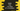# Kotlin program to find the positive value of negative numbers## Introduction :

In this tutorial, we will learn how to convert one negative number to positive in Kotlin. We will learn two different ways to do that.

### Method 1: Using abs() :

Similar to Java Math.abs, Kotlin provides one abs method defined in kotlin.math.abs. This method can be used to get the absolute value, i.e. the positive value of a negative number. For example :

``````import kotlin.math.abs

fun main() {
val givenNos = intArrayOf(-10, 10, 0, -123, -1000)

givenNos.forEach {
println("\$it -> \${abs(it)}")
}
}
``````

It will print the below output :

``````-10 -> 10
10 -> 10
0 -> 0
-123 -> 123
-1000 -> 1000
``````

### Method 2: Using absoluteValue :

absoluteValue property is define to return the absolute value of a number. You can also use it instead of abs() method. For example :

``````import kotlin.math.absoluteValue

fun main() {
val givenNos = intArrayOf(-10, 10, 0, -123, -1000)

givenNos.forEach {
println("\$it -> \${it.absoluteValue}")
}
}
``````

It will print the same output.

``````-10 -> 10
10 -> 10
0 -> 0
-123 -> 123
-1000 -> 1000
``````